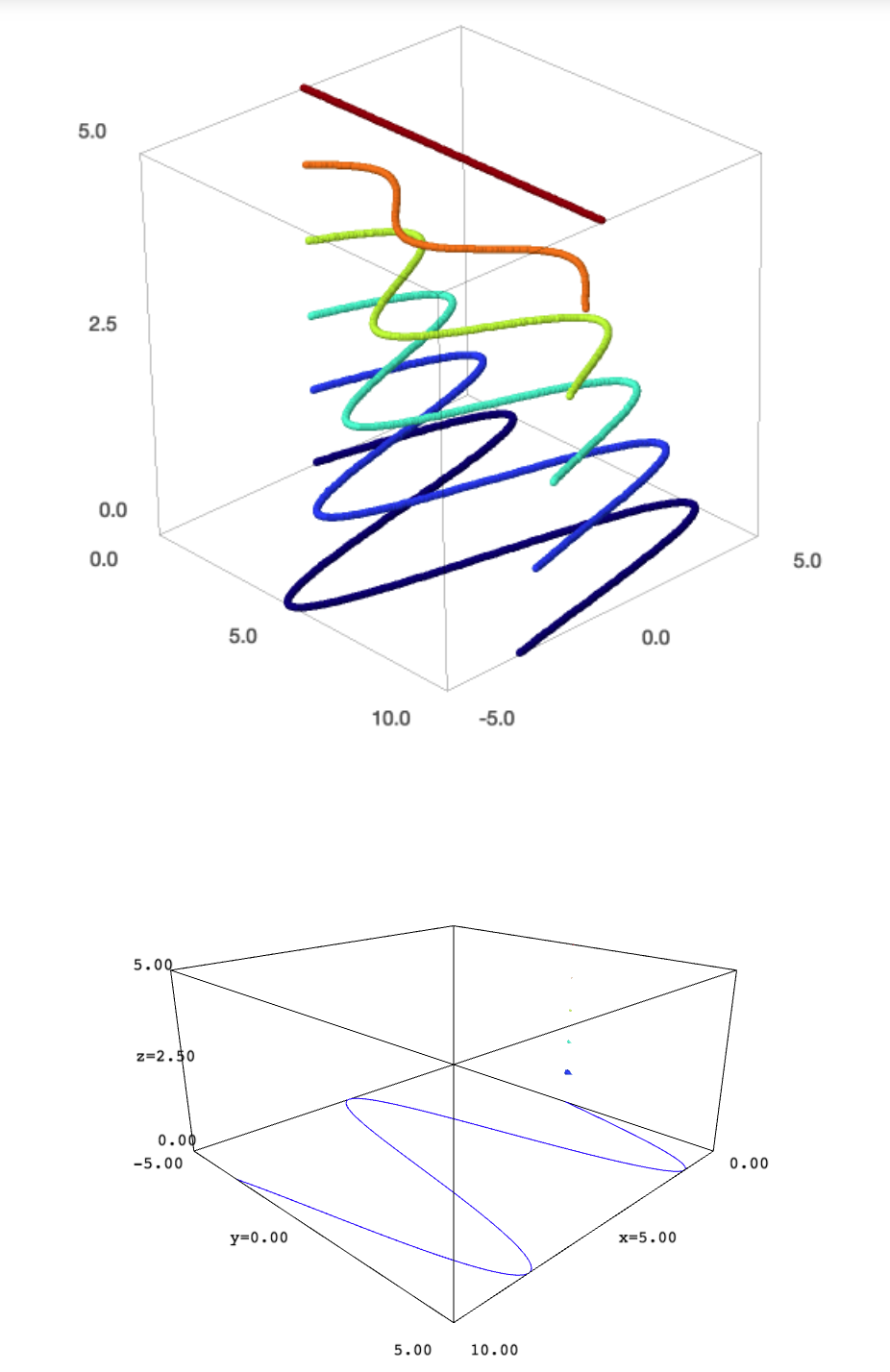# plot3d method does not properly work with threejsThe plot3d method allows to embed 2D objects in 3D graphics. It seems to work properly with the jmol viewer. However, it fails when the viewer is threejs. For example, the code

p = sum(plot((5-k)*sin(x), 0, 10, thickness=10,
color=colormaps.jet(50*k)).plot3d(z=k) for k in range(6))
show(p, viewer="jmol")
show(p, viewer="threejs")


yields this result:The first graphic, corresponding to jmol, is correct. The second graphic, produced by threejs, is completely wrong.

edit retag close merge delete

Doing sum(parametric_plot3d([x,(5-k)*sin(x), k], (x, 0, 10)) for k in range(6)) gives the same result in both viewers, but threejs doesn't seem to like this thickness or color.

Certainly it is possible to search workarounds. But what I just wanted is to draw attention to the fact that the default viewer does not behave properly in the described situation. The example given in the docs (search for plot3d in this page) does not longer work. Perhaps somebody who knows to open trac tickets could add one on this issue.

sagemath notebook 9.0 ,OS Ubuntu 18.04 yes threejs do not care about color.

a = vector([1, 2,5])
b = vector([4, 3,3])
plot(a, color='red')
P=plot(a, color='red') + plot(b, color='blue') + plot(a + b, color='black')
show(P, viewer="jmol")
show(P, viewer="threejs")


moreover both viewer do not accept 2d vectors

a = vector([1, 2])
b = vector([4, 3])
plot(a, color='red')
P=plot(a, color='red') + plot(b, color='blue') + plot(a + b, color='black')
show(P, viewer="jmol")
show(P, viewer="threejs")


matplotlib() got an unexpected keyword argument 'viewer'

The issue reported in the original question is fixed in the release candidate of SageMath 9.1 (to be out soon).